## Graphs: Other Trigonometric Functions

The tangent is an odd function because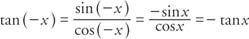The tangent has a period of π because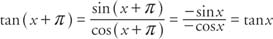The tangent is undefined whenever cos x = 0. This occurs when x = qπ/2, where q is an odd integer. At these points, the value of the tangent approaches infinity and is undefined. When graphing the tangent, a dashed line is used to show where the value of the tangent is undefined. These lines are called asymptotes. The values of the tangent for various angle sizes are shown in Table 1 .The graph of the tangent function over the interval from 0 to π/2 is as shown in Figure 1Figure 1
A portion of the tangent function.

The tangent is an odd function and is symmetric about the origin. The graph of the tangent over several periods is shown in Figure  2 . Note that the asymptotes are shown as dashed lines, and the value of the tangent is undefined at these points.Figure 2
Several periods of the tangent function.

The cotangent is the reciprocal of the tangent, and its graph is shown in Figure  3. Note the difference between the graph of the tangent and the cotangent in the interval from 0 to π/2.Figure 3
A portion of the cotangent function.

As shown in Figure 4, in the graph of the cotangent, the asymptotes are located at multiples of π.Figure 4
Several periods of the cotangent function.

Because the graphs of both the tangent and cotangent extend without bound both above and below the x‐axis, the amplitude for the tangent and cotangent is not defined.

The general forms of the tangent and cotangent functions are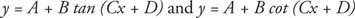The variables C and D determine the period and phase shift of the function as they did in the sine and cosine functions. The period is π/ C and the phase shift is |D/C|. The shift is to the right if | D/C | < 0, and to the left if | D/C | > 0. The variable B does not represent an amplitude because the tangent and cotangent are unbounded, but it does represent how much the graph is “stretched” in a vertical direction. The variable A represents the vertical shift.

Example 1: Determine the period, phase shift, and the location of the asymptotes for the function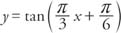and graph at least two complete periods of the function.

The asymptotes can be found by solving Cx + D = π/2 and Cx + D = −π/2 for X.The period of the function isThe phase shift of the function isBecause the phase shift is positive, it is to the left (Figure  5).Figure 5
Phase shift of the tangent function.

The amplitude is not defined for the secant or cosecant. The secant and cosecant are graphed as the reciprocals of the cosine and sine, respectively, and have the same period (2π). Therefore, the phase shift and period of these functions is found by solving the equations Cx + D = 0 and Cx + D = 2π for x.

Example 2: Determine the period, phase shift, and the location of the asymptotes for the function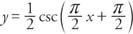and graph at least two periods of the function.

The asymptotes can be found by solving Cx + D = 0, Cx + D = π, and Cx + D = 2π for x.The period of the function isThe phase shift of the function is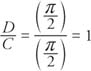Because the phase shift is positive, it is to the left.

The graph of the reciprocal function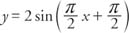is shown in Figure 6 . Graphing the sine (or cosine) can make it easier to graph the cosecant (or secant).